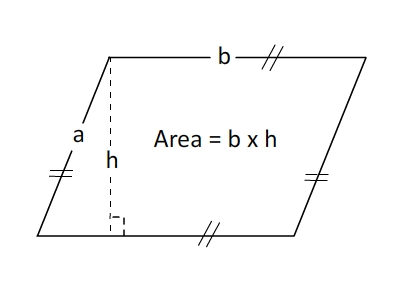# Parallelogram Area and Perimeter Calculator

Parallelogram Area Calculator tool is used to calculate area, perimeter and length of the diagonal of a parallelogram.## What is a Parallelograms ?

Like squares and rectangles, parallelograms are quadrilaterals: they have four sides and four interior angles. In a parallelogram those angles are not right angles, but the opposite sides must still be parallel to each other. The word "parallelogram" comes from the Greek word "parallelogrammon" (bounded by parallel lines). Rectangles, rhombuses, and squares are all parallelograms.

#### Perimeter of a Parallelogram

The perimeter of a parallelogram is the sum of its four sides. Since a parallelogram has two equal short sides (width, a ) and two equal long sides (base, b ), Perimeter=2a + 2b

#### Area of a Parallelogram

The area of a parallelogram is equal to its base (another name for length) times its height. Its height is not the same as its width: height is measured by a vertical line perpendicular (at right angles to) the base. Area=b * h

#### Characterizations of Parallelogram

A simple (non self-intersecting) quadrilateral is a parallelogram if and only if any one of the following statements is true:

• Two pairs of opposite sides are equal in length
• Two pairs of opposite angles are equal in measure
• The diagonals bisect each other
• One pair of opposite sides are parallel and equal in length
• Adjacent angles are supplementary
• Each diagonal divides the quadrilateral into two congruent triangles
• The sum of the squares of the sides equals the sum of the squares of the diagonals. (This is the parallelogram law)
• It has rotational symmetry of order 2
• It has two lines of symmetry

#### Properties of a Parallelogram

• Opposite sides of parallelogram are parallel.
• Any line through the midpoint of a parallelogram bisects the area.
• Parallelograms are quadrilaterals.